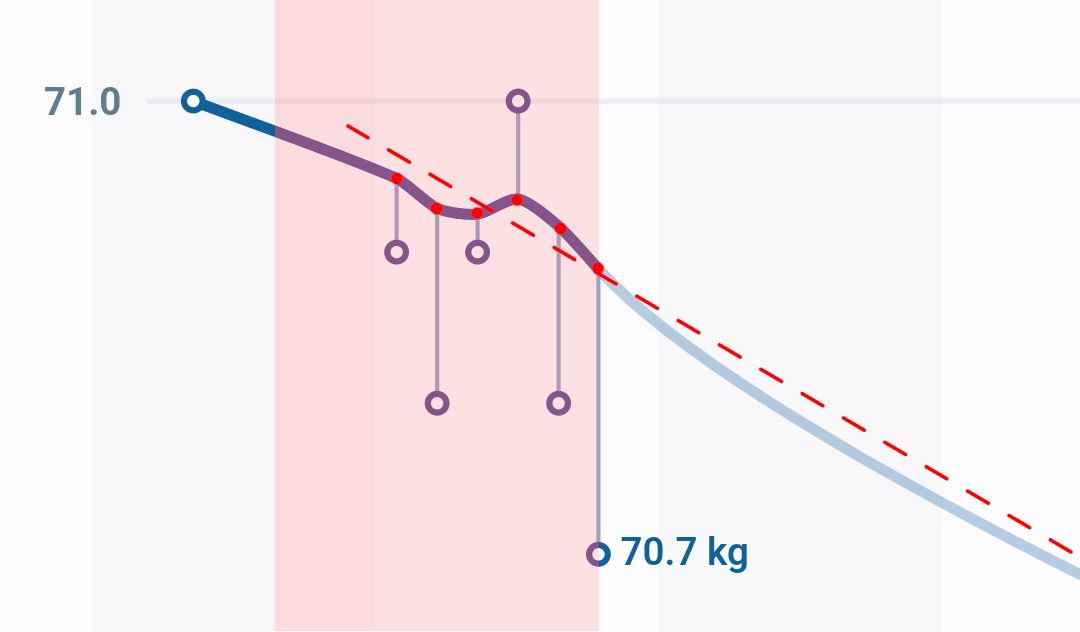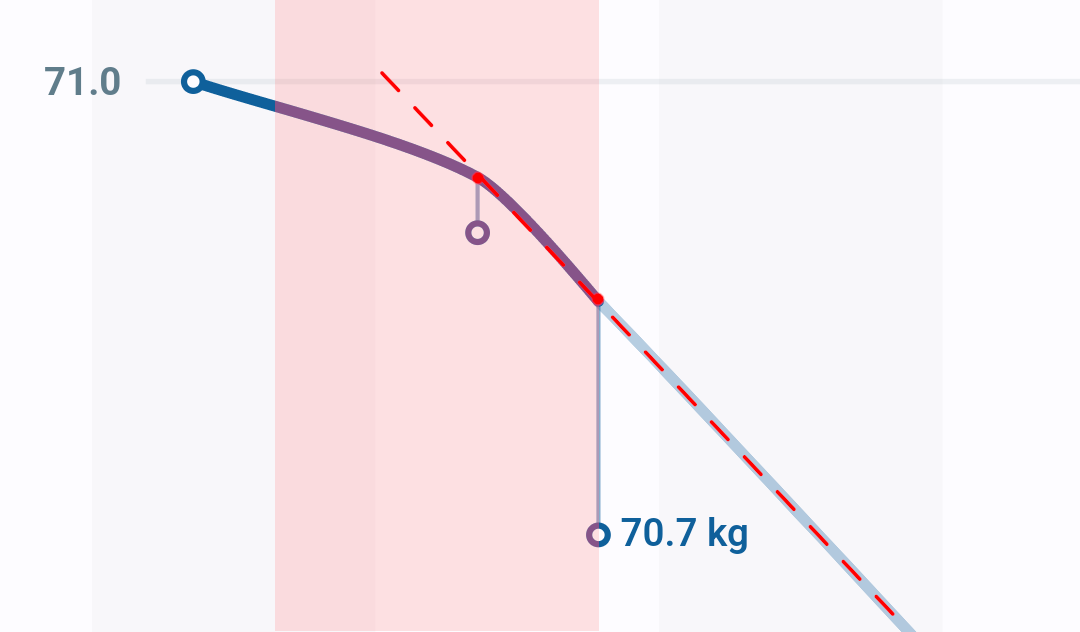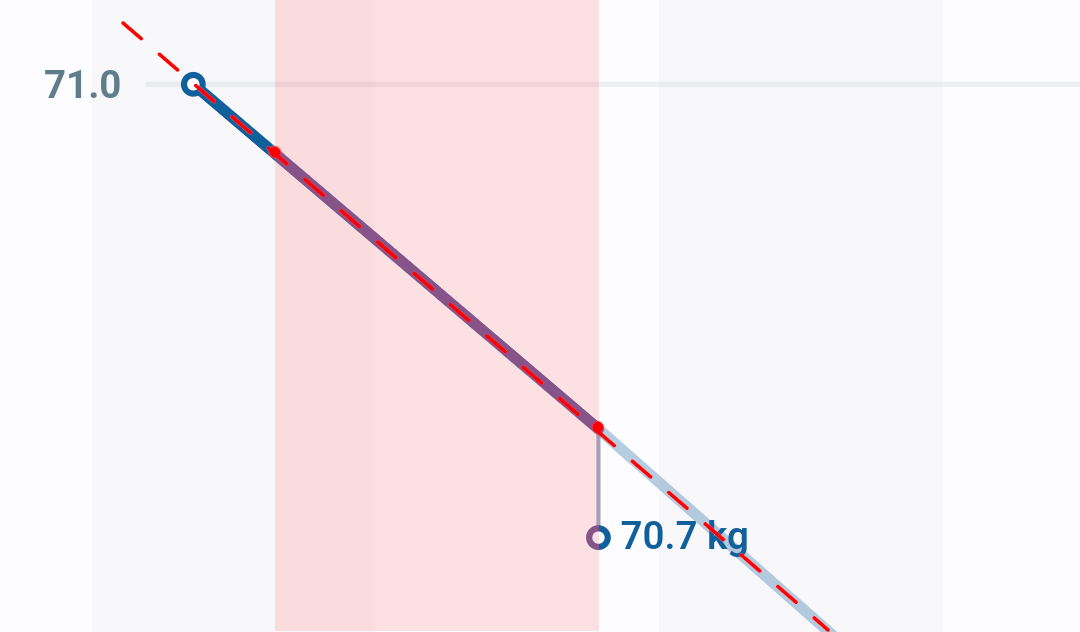# How forecasts are calculated

### Introduction

The forecast in Libra is an attempt at predicting the evolution of your weight based on the recent history.

The quality of the forecast is heavily dependent on the quality of the available data, but even the best forecast is only a rough estimation.

Past performance is no guarantee of future results

### Calculation

In order to calculate the forecast, Libra takes all the entries inside the forecast window, which is configurable in Advanced Preferences and defaults to 7 days.

A best-fit line is then computed using simple linear regression over the trend values for each entry in the window, with the resulting slope representing the rate of weight change. This makes it easy to calculate a forecast weight for a given date, or the date at which a given weight will be reached e.g. goal weight.#### Special cases

1. If there are only two values within the forecast window, Libra reverts to simple linear extrapolation of the line between the trends of both values.2. If there is only one value, Libra interpolates the trend value at the start of the forecast window and uses that for linear extrapolation.• The use of the trend instead of the actual weight values is due to the noisy nature of the latter. Using pre-smoothed values avoids the forecast changing substantially on each new entry. Naturally this means that the forecast is affected by the trend smoothing configuration.

• Libra’s forecast line only extends three months in the future. This was determined experimentally to be a happy medium between showing useful information while acknowledging that there will be variations in fitness and nutritional plans over longer periods of time.

• The forecast line in Libra’s chart is drawn as a curve that continues from the trend line, and then smoothly points towards the predicted weight.
This can be confusing if the forecast line doesn’t cross your goal weight at the mathematically-predicted date: in those cases Libra trades mathematical precision for a more pleasant graphical interface.